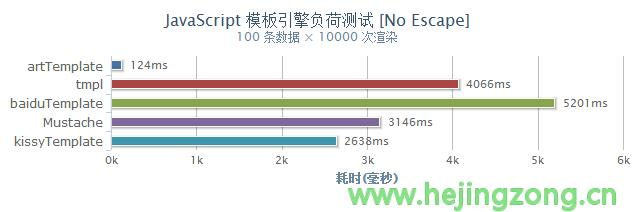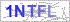## JavaScript模板引擎介绍、原理、实现

标签：JavaScript,模板引擎,artTemplate
修改 | 阅读（1015）| 评论（0）

### 什么是JavaScript引擎

```var html='<ul>';
for(var i=0;i<users.length;i++){
html+='<li><a href=">'+users[i].url+'">'+users[i].name+'</a>';
}
html+='</ul>';
document.getElementById('results').innerHTML=html;```

```<ul>
<% for ( var i = 0; i < users.length; i++ ) { %>
<li><a href="<%=users[i].url%>"><%=users[i].name%></a></li>
<% } %>
</ul>```

```<ul>
<li><a href="url1">name1</a></li>
<li><a href="url2">name2</a></li>
<li><a href="rul3">name3</a></li>
</ul>```

### javascript 模板引擎基本原理

```<ul>
<% for ( var i = 0; i < users.length; i++ ) { %>
<li><a href="<%=users[i].url%>"><%=users[i].name%></a></li>
<% } %>
</ul>```

```var p=[];
p.push('<ul>');
for(var i=0;i<users.length;i++){ //javascript语句执行
p.push('<li><a href="'); //html语句拼接
p.push(users[i].url); //javascript变量置换后拼接
p.push('">');
p.push(users[i].name);
p.push('</a></li>');
}
p.push('</ul>');```

```<script type="text/html" id="user_tmpl">
<ul>
<% for ( var i = 0; i < users.length; i++ ) { %>
<li>
<a href="<%=users[i].url%>"><%=users[i].name%></a>
</li>
<% } %>
</ul>
</script>```

```<%=xxx%>    →    ');p.push(xxx);p.push('
<%          →    ');
%>          →    p.push('```

```p.push('<ul>');
for(var i=0;i<users.length;i++){
p.push('<li><a href="');
p.push(users[i].url);
p.push('">');
p.push(users[i].name);
p.push('</a></li>');
}
p.push('</ul>');```

1.把<%=xxx%> 替换为 ');p.push(xxx);p.push('

`html=html.replace(/<%=(.*?)%>/g,"');p.push(xxx);p.push('");`

2.把<%替换为 ');

`html=html.replace(/<%/g,"');");`

3.把%> 替换为 p.push('

`html=html.replace(/%>/g,"p.push('");`

```function tmpl(id,data){
var html=document.getElementById(id).innerHTML;
var result="var p=[]; p.push('"
+html.replace(/<%=(.*?)%>/g,"');p.push(xxx);p.push('")
.replace(/<%/g,"');")
.replace(/%>/g,"p.push('")
+" ');";
}```

```var result="
var p=[];
p.push('<ul>');
for(var i=0;i<users.length;i++){
p.push('<li><a href="');
p.push(users[i].url);
p.push('">');
p.push(users[i].name);
p.push('</a></li>');
}
p.push('</ul>');"```

```function fn(data){
console.log(data);
}```

`var fn = new Function(arg1, arg2, ..., argN, function_body)`

```function tmpl(id,data){
var html=document.getElementById(id).innerHTML;
var result="var p=[];with(obj){p.push('"
+html.replace(/[\r\n\t]/g," ")
.replace(/<%=(.*?)%>/g,"');p.push(\$1);p.push('")
.replace(/<%/g,"');")
.replace(/%>/g,"p.push('")
+"');}return p.join('');";
var fn=new Function("obj",result);
return fn(data);
}```

### 例子

```<!DOCTYPE html>
<html>
<title>模板</title>
<body>
<!--结果容器-->
<div id="results"></div>
<!--模板-->
<script type="text/html" id="user_tmpl">
<ul>
<% for ( var i = 0; i < users.length; i++ ) { %>
<li><a href="<%=users[i].url%>"><%=users[i].name%></a></li>
<% } %>
</ul>
</script>
<script type="text/javascript">
//模板数据
var results = document.getElementById("results");
var users=[
{"name":"列表1", "url":"http://www.hejingzong.cn"},
{"name":"列表2", "url":"http://www.hejingzong.cn"},
{"name":"列表3", "url":"http://www.hejingzong.cn"}
];
//模板函数
function tmpl(id,data){
var html=document.getElementById(id).innerHTML;
var result="var p=[];with(obj){p.push('"
+html.replace(/[\r\n\t]/g," ")
.replace(/<%=(.*?)%>/g,"');p.push(\$1);p.push('")
.replace(/<%/g,"');")
.replace(/%>/g,"p.push('")
+"');}return p.join('');";
var fn=new Function("obj",result);
return fn(data);
}
//输出结果
results.innerHTML = tmpl("user_tmpl", users);
</script>
</body>
</html>```

### 不足之处

1、性能：模板引擎渲染的时候依赖 Function 构造器实现，Function 与 eval、setTimeout、setInterval 一样，提供了使用文本访问 javascript 解析引擎的方法，但这样执行 javascript 的性能非常低下。

2、调试：由于是动态执行字符串，若遇到错误调试器无法捕获错误源，导致模板 BUG 调试变得异常痛苦。在没有进行容错的引擎中，局部模板若因为数据异常甚至可以导致整个应用崩溃，随着模板的数目增加，维护成本将剧增。

### artTemplate 介绍

artTemplate 是新一代 javascript 模板引擎，它采用预编译方式让性能有了质的飞跃，并且充分利用 javascript 引擎特性，使得其性能无论在前端还是后端都有极其出色的表现。在 chrome 下渲染效率测试中分别是知名引擎 Mustache 与 micro tmpl 的 25 、 32 倍。### artTemplate 高效的秘密

1、预编译

```var render=function(\$data){
var content=\$data.count,\$out='';
\$out += '<h3>';
if(typeof count === 'string'){
\$out += count;
}
\$out += '</h3>';
return \$out;
};
render({content:'逍遥乐网博客'});```

2、更快的字符串相加方式

https://github.com/aui/artTemplate/

http://aui.github.com/artTemplate/

Juicer —— 一个Javascript模板引擎的实现和优化

QQ登录(无需注册直接登录可进行回复)